# Multiple Choice Questions and Answers on Automatic Control System

## Multiple Choice Questions and Answers on Automatic Control System

Q1. From the noise point of view, bandwidth should be

a) large
b) not too large
c) should be as large as possible
d) infinite

Q2. In a two phase ac servomotor rotor resistance is R and rotor reactance is X. The speed curve will be linear if

a) (X/R)<<1
b) (X/R) >>1
c) (X/R)=1
d) X²=R

Q3. A stepper motor is

a) a two phase induction motor
b) is an electromagnetic transducer used to convert an angular position of shaft into electrical signal
c) is an electromechanical device which actuates a train of step angular movements in response to a train of input pulses on one to one basis
d) is a kind of rotating amplifier

Q4. First column elements of Routh’s tabulation are 3, 5, -3/4, 1/2, 2. It means

a) there is one root in left half s plane
b) there are two roots in left half s plane
c) there are two roots in right half s plane
d) there is one root in right half s plane

Q5. When a unit step voltage drives a lag network the output

a) remains constant at unit step value
b) increases exponentially from zero to final value
c) decreases exponentially from 1 to 0
d) none of the above

Q6. A system has its two poles on the negative real axis and one pair of poles lies on jω axis. The system is

a) stable
b) unstable
c) limitedly stable
d) none of the above

Q7. Bellows converts

a) pressure difference into displacement
b) pressure difference into voltage
c) displacement into pressure difference
d) none of the above

Q8. Assertion (A): The steady state response, of a stable, linear, time invariant system, to sinusoidal input depends on initial conditions.

Reason (R): Frequency response, in steady state, is obtained by replacing s in the transfer function by jω

a) Both A and R are correct and R is correct explanation of A
b) Both A and R are correct but R is not correct explanation of A
c) A is correct but R is wrong
d)R is correct but A is wrong

Q9. The phase margin and damping ratio have no relation.

a) True
b) False

Q10. For the transport lag G(jω) = e-jωT, the magnitude is always equal to

a) 0
b) 1
c) 10
d) 0.5

Q11. The log magnitude curve for a constant gain K is a

a) horizontal straight line
b) horizontal straight line of magnitude 20 log K decibels
c) an inclined line having slope K
d) an inclined line having slope -K

Q12. A lag compensator is essentially a

a) low pass filter
b) high pass filter
c) band pass filter
d) none of the above

Q13. Stepper motors find applications in

a) X-Y plotters
b) numerically controlled machining equipment
c) printers
d) all of the above

Q14. For type 2 system, the magnitude and phase angle of the term (jω)2 in the denominator, at ω = 0, are respectively

a) 0 and – 90°
b) 0 and + 90°
c) infinity and – 180°
d) infinity and + 180°

Q15. In an integral controller

a) the output is proportional to input
b) the rate of change of output is proportional to input
c) the output is proportional to rate of change of input
d) none of the above

Q16.In Bode diagram (log magnitude plot) the factor 1/jω in the transfer function gives a line having slope

a) -20 dB per octave
b) -10 dB per octave
c) -6 dB per octave
d) -2 dB per octave

Q17. Bode magnitude plot is drawn between

a) magnitude of network function and ω
b) dB magnitude and log ω
c) dB magnitude and ω
d) loge (magnitude) and log ω

Q18. The system in the given figure, has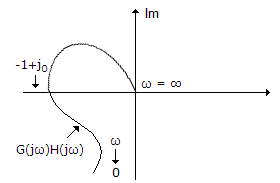a) good gain and phase margin
b) poor gain and phase margin
c) good gain margin but poor phase margin
d) poor gain margin but good phase margin

Q19. For very low frequencies, v0/vi in the given figure equals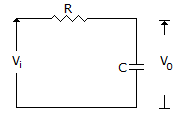a) 1
b) 0
c) 0.5
d) infinity

Q20. In a minimum phase system

a) all poles lie in the left half plane
b) all zeros lie in the left half plane
c) all poles lie in the right half plane
d) all except one pole or zero lie in the left half plane

Q21. For the control system in the given figure, the value of K for critical damping is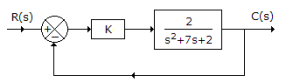a) 1
b) 5.125
c) 6.831
d) 12

Q22. In the given figure, if R = XC, voltage gain is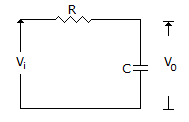a) 0 dB
b) -3 dB
c) 3 dB
d) 10 dB

Q23.  In the given figure the input is x(t) = A sin ωt. The steady state output y(t) =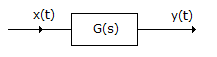a) A sin (ωt + φ) where φ = tan-1 |G(jω)|
b) |G(jω)| A sin [ωt + ∠G(jω)]
c) |G(jω)| A sin [2ωt + ∠G(jω)]
d) A G(jω) sin [ωt + ∠G(jω)]

Q24. The compensator of the given figure is a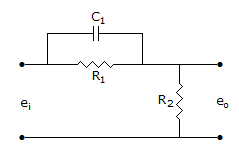a) lag compensator
d) none of the above

Q25. In the given figure, of potentiometer V0 = Vi (R0/Ri) only when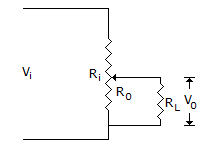a) RL = 0
b) RL = ∞
c) RL is neither low nor high
d) RL is low but not zero

Q26. For the system of the given figure, the damping ratio of closed loop poles is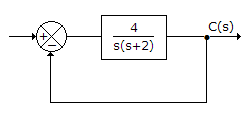a) 1.5
b) 1
c) 0.5
d) 0.25

Q27. The first column of a Routh array is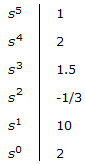How many roots of the corresponding characteristic equation are in left half s-plane?

a) 2
b) 3
c) 4
d) 5

Q28. The entries in the first column of Routh array of a fourth order are 5, 2, – 0.1, 2, 1. The number of poles in the right half plane are

a) 1
b) 2
c) 3
d) 4

Q29. In Bode diagram (log magnitude plot) the factor (jω)n in the transfer function gives a line having slope

Q30. The magnitude of transport lag factor is always zero.

a) True
b) False

Q31. For a quadratic factor in transfer function the phase angle is not a function of damping factor.

a) True
b) False

Q32. The current rating of dc tachogenerator is usually

a) small
b) high
c) medium
d) either (b) or (c)

Q33. For the compensator in the given figure the maximum phase lead is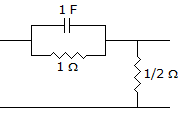a) sin-1 (1/3)
b) 30°
c) 45°
d) 60°

Q34. Assertion (A): Positive feedback is not used in any circuit.

Reason (R): Positive feedback leads to instability.

a) Both A and R are correct and R is correct explanation of A
b) Both A and R are correct but R is not correct explanation of A
c) A is correct but R is wrong
d) R is correct but A is wrong

Q35. If the Nyquist plot does not encircle the (-1 + j0) point the system is always stable.

a) True
b) False

Q36. Assertion (A): When the phase margin of a control system is improved using a lead network, noise transmission increases.

Reason (R): Phase lead compensation increases bandwidth.

a) Both A and R are correct and R is correct explanation of A
b) Both A and R are correct but R is not correct explanation of A
c) A is correct but R is wrong
d) R is correct but A is wrong

Q37. In liquid flow system the capacitance of a water tank is defined as

a) change in liquid stored / change in head
b) change in head / change in liquid stored
c) change in flow rate / change in head
d) none of the above

Q38. For a first order system having transfer function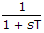, the unit ramp response is

a) T(1 – e-t/T)
b) t – T + T e-t/T
c) t + T + T e-t/T
d) t + 2T e-t/T

Q39. In a second order system, the time constant t of exponential envelopes depends

a) only on damping factor
b) only on natural frequency
c) both on damping factor and natural frequency
d) neither on damping factor nor on natural frequency

Q40. The maximum shift which can be provided by a lead compensator with transfer function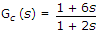is

a) 15°
b) 30°
c) 45°
d) 60°

Q41. The network of the given figure is a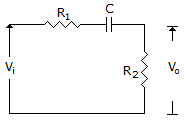a) lag network
d) any of the above

Q42. For a second order system, the position of poles is in the shaded region shown in the given figure. The variation in damping factor isa) from 0 to 1
b) from about 0.3 to 0.7
c) from 1 to 5
d) from 0 to 3

Q43. For a factor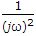in the transfer function, the log magnitude plot

a) is a straight line with slope 0 dB/decade
b) is a straight line with slope 20 dB/decade
c) is a straight line with slope – 20 dB/decade
d) is a straight line with slope – 40 dB/decade

Q44. The frequency at which magnitude of closed loop frequency response is 3 dB below its zero frequency value is called critical frequency.

a) True
b) False

Q45. The transfer function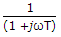has the characteristics of high pass filter.

a) True
b) False

Q46. The system of the given figurea) has two poles and one zero in left half plane
b) has two poles and one zero in right half plane
c) has two poles in left half plane and zero in right half plane
d) none of the above

Q47. If phase angle of open loop transfer function becomes – 180° at frequency ω1, then gain margin is equal to

a) |G(jω1)|
b) 1/ |G(jω1)|
c) 1+ |G(jω1)|
d) 1/ 1+|G(jω1)|

Q48. Assertion (A): The time response of the system with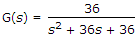will not have any overshoot for unit step input.

Reason (R): A critically damped system does not have any overshoot to a unit step input.

a) Both A and R are correct and R is correct explanation of A
b) Both A and R are correct but R is not correct explanation of A
c) A is correct but R is wrong
d) R is correct but A is wrong

Q49. Lag compensation increases bandwidth of the system.

a) True
b) False

Q50. The polar plot of the given figure is for the terma) (jω)2
b) 1 + (jω)2
c) 1/ (jω)2
d) 1/(1 + jω)

Q51. For the unity feed back system of the given figure, the closed loop transfer function is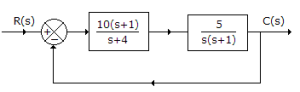a) 50/ s²+4s+50
b) 50/s+50
c) 50/s(s+4)
d) 50/s²+50

Q52. The effect of negative feedback on distortion and bandwidth because

a) both are decreased
b) both are increased
c) distortion is reduced and bandwidth is increased
d) distortion is increased and bandwidth is decreased

Q53. The polar plot of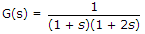a) does not cross real axis
b) crosses real axis
c) crosses real axis at ω = 0.7 rad/sec
d) crosses real axis at ω = 1.4 rad/sec

Q54. In a second order undamped system, the poles are on

a) positive real axis
b) imaginary axis
c) negative real axis
d) either (a) or (c)

Q55. For the system of the given figure, the undamped natural frequency of closed loop poles is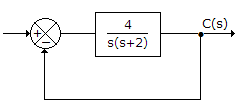Q56. An open loop system has a forward path transfer function (42.25)/s(s + 6.5). The unit step response of the system starting from rest will have its maximum value at a time equal to

a) 0 sec
b) 0.56 sec
c) 5.6 sec
d) infinity

Q57. The polar plot in the given figure is for the term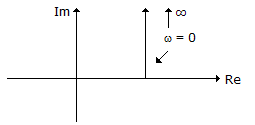a) jωT
b) 1 + jωT
c) 1/ jωT
d) 1/(1 + jωT)

Q58. The compensator in the given figure is a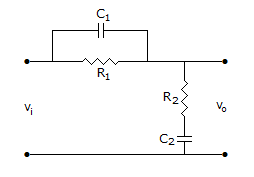a) lag compensator
d) none of the above

Q59. If G(s) H(s) =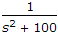, the closed loop poles are on

a) negative real axis
b) jω axis
c) right half plane
d) either (a) or (b)

Q60. For the block diagram of the given figure, the equation describing system dynamics is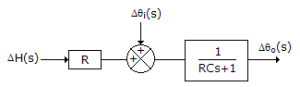a) (1 + RCs) Δθ0(s) = Δθi(s) + R Δ H(s)
b) (1 + RCs) [ΔH(s)] = Δθ(s) – Δθ0(s)
c) (1 + RCs) Δθ0(s) = RΔ H(s) – Δ θi(s)
d) (1 + RCs) Δθi(s) = Δ H(s)[R] – Δθ0(s)

Q61. For the given figure C(s)/R(s)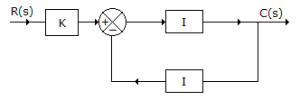a) K
b) 0.5 K
c) 2 K
d) 0.25 K

Q62. Modelling error may occur due to

a) neglecting the nonlinear characteristics of plant
b) inaccuracy of parameters
c) change of plant characteristics with time
d) any of the above

Q63. In the given figure, P = 3 kg force. Then X(s) =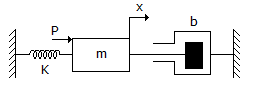a) 3/ (ms +b +K)
b) 3/ (ms² +bs +K)
c) 3/ s(ms² +bs +K)
d) 3/ s² (ms² +bs +K)

Q64. In Bode diagrams an octave is a frequency band from

a) ω1 to 10 ω1
b) ω1 to 8 ω1
c) ω1 to 4 ω1
d) ω1 to 2 ω1

Q65. In position control system the device used for providing rate feedback voltage is

a) potentiometer
b) synchro-transmitter
c) synchro-transformer
d) tacho generator

Q66. Derivative control action is effective?

a) Only during steady state periods
b) Only during transient state periods
c) During both steady state and transient state periods
d) Either during steady state or during transient state periods

Q67. The response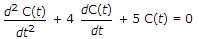is

a) undamped
b) underdamped
c) critically damped
d) oscillatory

Q68. For a first order system having transfer function, the unit step response is

a) 1 – et/T
b) e-t/T
c) –e-t/T – 1
d) 1 + e-t/T

Q69. Which of the following is not a necessary advantage of negative feedback system?

a) Feedback improves speed of response
b) Feedback improves stability
c) Feedback reduces effect of variations in system parameters
d) Feedback reduces the effect of external disturbances

Q70. A system has 12 poles and 2 zeros. Its high frequency asymptote in its magnitude plot has a slope of

Q71. The open loop transfer function of a unity feedback system is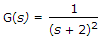The poles of closed loop system are at

a) -2, -2
b) -2, -1
c) – 2, ± j 1
d) – 2, 2

Q72. If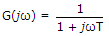the polar plot, as the frequency is varied from 0 to infinity is

a) a semicircle
b) a circle
c) a straight line
d) none of the above

Q73. Examine the following statements

1. Air and gases are compressible.
2. Air, gases and some liquids are compressible.
3. Oil can function as hydraulic fluid as well as lubricator.

Which of the above statements are correct?

a) 3 only
b) 2 and 3 only
c) 1 and 3 only
d) 2 only

Q74. Which control action is also called rate control?

a) Proportional
b) Derivative
c) Integral
d) Proportional plus integral

Q75. The gain margin for a stable system

a) has a positive decibel value
b) has a negative decibel value
c) has a large negative decibel value
d) none of the above

Q76. The signal flow graph of a system is shown in the given figure. The effect of disturbance TD can be reduced by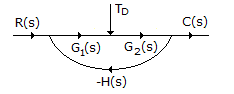a) increasing G2 (s)
b) decreasing G2 (s)
c) increasing G1 (s)
d) decreasing G1 (s)

Q77. For overdamped systems a quadratic factor in transfer function can be replaced by two first order factors with real poles

a) True
b) False

Q78. The gain margin of first and second order systems is infinite.

a) True
b) False

Q79. One advantage of using polar plot is that it depicts the frequency response characteristics over the entire frequency.

a) True
b) False

Q80. The force required to initiate motion between two contacting surfaces is called

a) coulomb friction
b) viscous friction
c) static friction
d) none of the above

Q81. Assertion (A): Derivative control action cannot be used alone.

Reason (R): Derivative control action is effective only during transient periods.

a) Both A and R are correct and R is correct explanation of A
b) Both A and R are correct but R is not correct explanation of A
c) A is correct but R is wrong
d) R is correct but A is wrong

Q82. The slope of log magnitude curve near the cut off frequency is called cut off rate.

a) True
b) False

Q83. The system in the given figure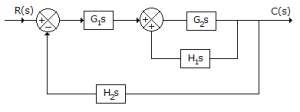a) will be unstable due to the presence of positive feedback in the inner loop
b) may be stabilized by the negative feedback outer loop
c) can never be stable
d) will always be stable

Q84. The units of thermal capacitance are

a) k cal/°C
b) °C/k cal
c) °C sec/k cal
d) none of the above

Q85. Nichols chart consists of M and N locii in log magnitude versus phase diagram.

a) True
b) False

Q86. If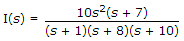, initial and final values of i(t) are

a) zero and 1
b) zero and 10
c) 10 and zero
d) 70 and 80

Q87. The given figure shows three Nyquist plots of G(s) H(s) = e-sT/[s(s + 1)] for different values of T. The curves 1, 2, 3 can bea) for T = 0, 0.5 and 1 respectively
b) for T = 1, 0.5 and 0 respectively
c) for T = 0.5, 1 and 0 respectively
d) for T = 0, 1 and 0.5 respectively

Q88. Consider the following statements:
The gain margin and phase margin of an unstable system may respectively be

1. positive, positive
2. positive, negative
3. negative, positive
4. negative, negative

Of these statements

a) 1, 4 are correct
b) 1 and 2 correct
c) 1, 2, 3 are correct
d) 2, 3, 4 are correct

Q89. A first order element yields almost exact duplication only for constant or slowly varying phenomenon.

a) True
b) False

Q90. For a stable system

a) both phase and gain margin are negative
b) both phase and gain margin are positive
c) phase margin is positive but gain margin is negative
d) gain margin is positive but phase margin is negative

Q91. If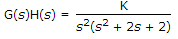the angle of departure of root locus at s = – 1 + j is

a) zero
b) 90°
c) -90°
d) -180°

Q92. The number of branches of root locus plot is equal to

a) the number of roots of characteristic equation
b) double the number of roots of characteristic equation
c) the number of roots of characteristic equation minus one
d) the number of roots of characteristic equation plus one

Q93. The action of bellows is similar to that of

a) mass
b) viscous friction
c) spring
d) none of the above

Q94. As the bandwidth increases, the cost of components

a) generally decreases
b) generally increases
c) may increase or decrease
d) does not change

Q95.  If Nyquist plot has a encirclement of (-1 + jo) point, the system is stable if

a) there are no poles of G(s) H(s) in right-half plane
b) there is one pole of G(s) H(s) in right-half plane
c) there is no zeros of G(s) H(s) in right-half plane
d) none of the above

Q96. A conditionally stable system has

a) one phase crossover frequency
b) two phase crossover frequencies
c) two or more phase crossover frequencies
d) one or more phase crossover frequencies

Q97. In a pneumatic bellows

a) the input surface, output surface and side walls are corrugated
b) the input surface and output surface are flat but the side walls are corrugated
c) the input surface and side walls are flat but the output surface is corrugated
d) the input surface and output surface are corrugated but the side walls are flat

Q98. If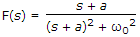then f(t) =

a) cos θ0t
b) sin ω0t
c) eat cos ω0t
d) e-at cos ω0t

Q99. When time constant T in any factor is varied, the shapes of log magnitude and phase angle curves remain the same.

a) True
b) False